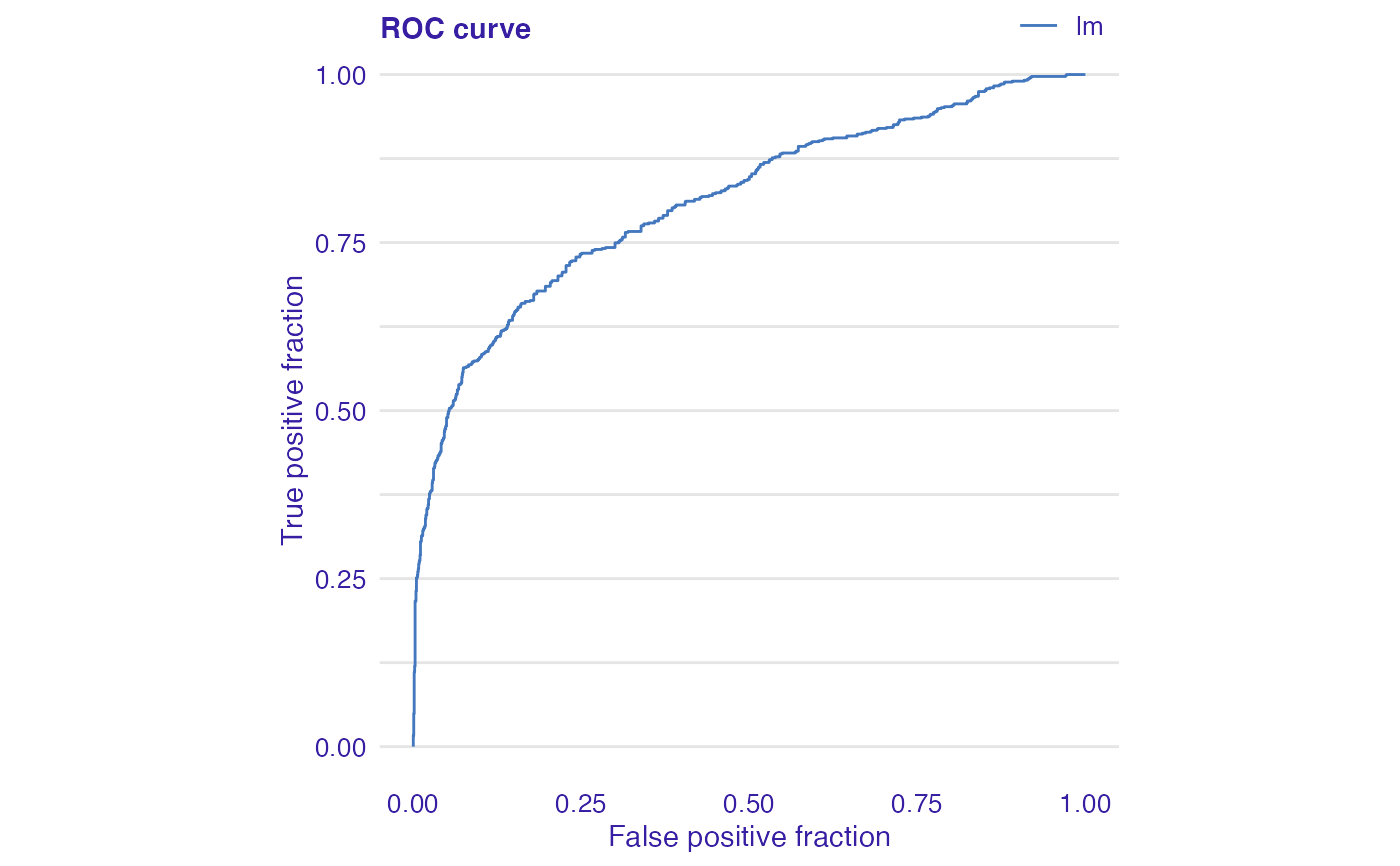Creates explanation of classification model.

Returns, among others, true positive rate (tpr), false positive rate (fpr), rate of positive prediction (rpp), and true positives (tp).

Created object of class auditor_model_evaluation can be used to plot Receiver Operating Characteristic (ROC) curve (plot plot_roc) and LIFT curve (plot plot_lift).

model_evaluation(object)

modelEvaluation(object)

## Arguments

object An object of class explainer created with function explain from the DALEX package.

## Value

An object of the class auditor_model_evaluation.

## Examples

data(titanic_imputed, package = "DALEX")

# fit a model
model_glm <- glm(survived ~ ., family = binomial, data = titanic_imputed)

glm_audit <- audit(model_glm,
data= titanic_imputed,
y = titanic_imputed\$survived)
#> Preparation of a new explainer is initiated
#>   -> model label       :  lm  (  default  )
#>   -> data              :  2207  rows  8  cols
#>   -> target variable   :  2207  values
#>   -> predict function  :  yhat.glm  will be used (  default  )
#>   -> predicted values  :  No value for predict function target column. (  default  )
#>   -> model_info        :  package stats , ver. 4.1.1 , task classification (  default  )
#>   -> predicted values  :  numerical, min =  0.008128381 , mean =  0.3221568 , max =  0.9731431
#>   -> residual function :  difference between y and yhat (  default  )
#>   -> residuals         :  numerical, min =  -0.9628583 , mean =  -2.569729e-10 , max =  0.9663346
#>   A new explainer has been created!
# validate a model with auditor
me <- model_evaluation(glm_audit)
me
#> Model label:  lm
#>
#>  True Positive Rate for cutoff 0.5: 0
#>
#>  False Positive Rate for cutoff 0.5: 0
plot(me)